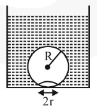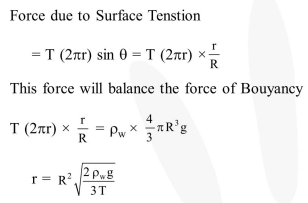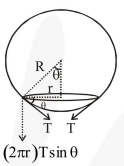Deepak Scored 45->99%ile with Bounce Back Crack Course. You can do it too!

# On heating water,

Question:

On heating water, bubbles being formed at the bottom of the vessel detatch and rise. Take the bubbles to be spheres of radius $R$ and making a circular contact of radius $r$ with the bottom of the vessel. If $\mathrm{r} \ll \mathrm{R}$, and the surface tension of water is $\mathrm{T}$, value of $r$ just before bubbles detatch is:(dencity of water is $\rho_{w}$ )1. $R^{2} \sqrt{\frac{\rho_{w} g}{T}}$

2. $\mathrm{R}^{2} \sqrt{\frac{3 \rho_{w} \mathrm{~g}}{\mathrm{~T}}}$

3. $\mathrm{R}^{2} \sqrt{\frac{2 \rho_{\mathrm{w}} \mathrm{g}}{3 \mathrm{~T}}}$

4. $R^{2} \sqrt{\frac{\rho_{\mathrm{w}} \mathrm{g}}{6 \mathrm{~T}}}$

Correct Option: 3,

Solution: